# How to Calculate and Solve for Impulse | Motion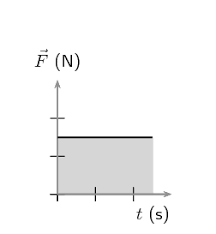The image above represents impulse.

To compute for impulse, two essential parameters are needed and these parameters are force (f) and time (t).

The formula for calculating impulse:

I = Ft

Where;

I = Impulse
F = Force
t = Time

Let’s solve an example;
Given that the force is 28 and the time is 14. Find the impulse?

This implies that;

F = Force = 28
t = Time = 14

I = Ft
I = 28 x 14
I = 392

Therefore, the impulse is 392 Ns.

Calculating the Force when the Impulse and the Time is Given.

F = I / t

Where;

F = Force
I = Impulse
t = Time

Let’s solve an example;
Given that the impulse is 60 and the time is 10. Find the force?

This implies that;

I = Impulse = 60
t = Time = 10

F = I / t
F = 60 / 10
F = 6

Therefore, the force is 6 N.

Calculating the Time when the Impulse and the Force is Given.

t = I / F

Where;

t = Time
I = Impulse
F = Force

Let’s solve an example;
Given that the force is 20 and the impulse is 32. Find the time?

This implies that;

I = Impulse = 32
F = Force = 20

t = I / F
t = 32 / 20
t = 1.6

Therefore, the time is 1.6 s.

Nickzom Calculator – The Calculator Encyclopedia is capable of calculating the impulse.

To get the answer and workings of the impulse using the Nickzom Calculator – The Calculator Encyclopedia. First, you need to obtain the app.

You can get this app via any of these means:

To get access to the professional version via web, you need to register and subscribe for NGN 1,500 per annum to have utter access to all functionalities.
You can also try the demo version via https://www.nickzom.org/calculator

Apple (Paid) – https://itunes.apple.com/us/app/nickzom-calculator/id1331162702?mt=8
Once, you have obtained the calculator encyclopedia app, proceed to the Calculator Map, then click on Motion under Physics.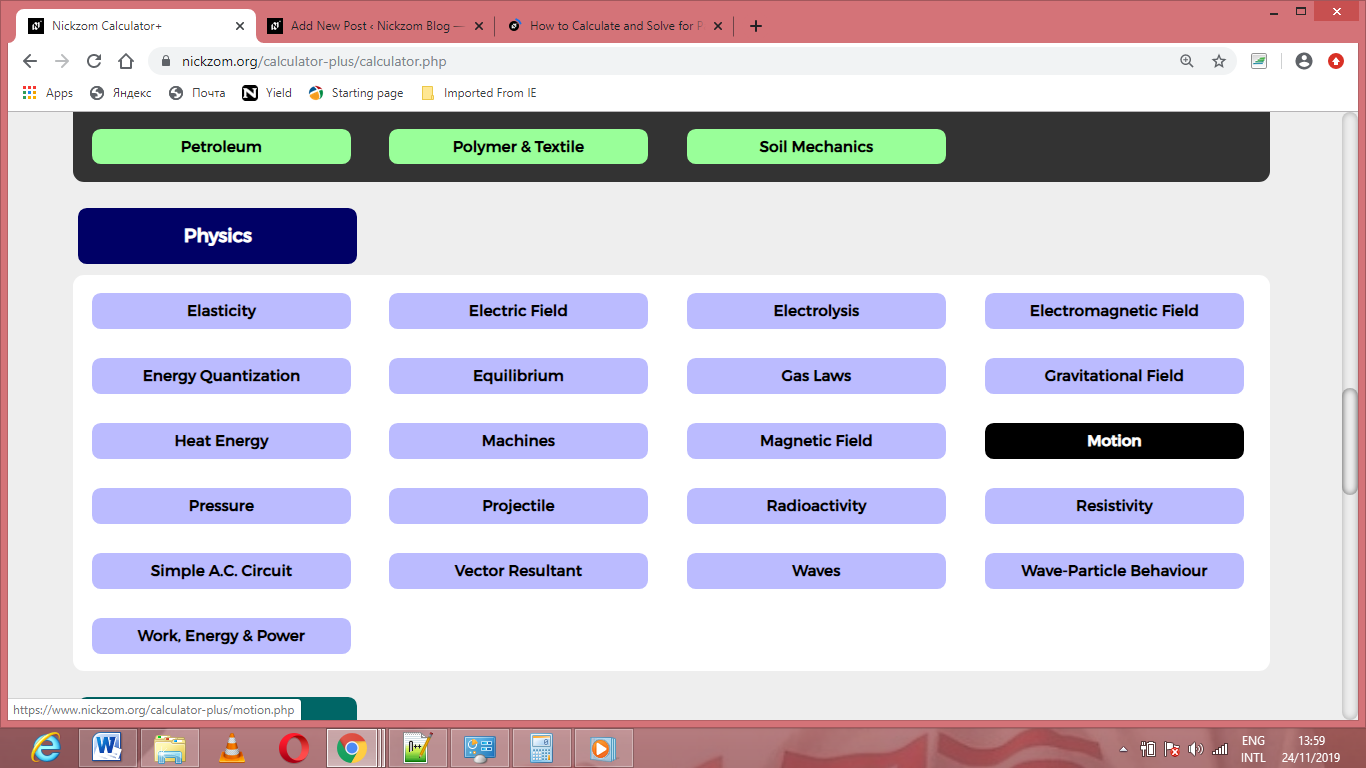Now, Click on Impulse under Motion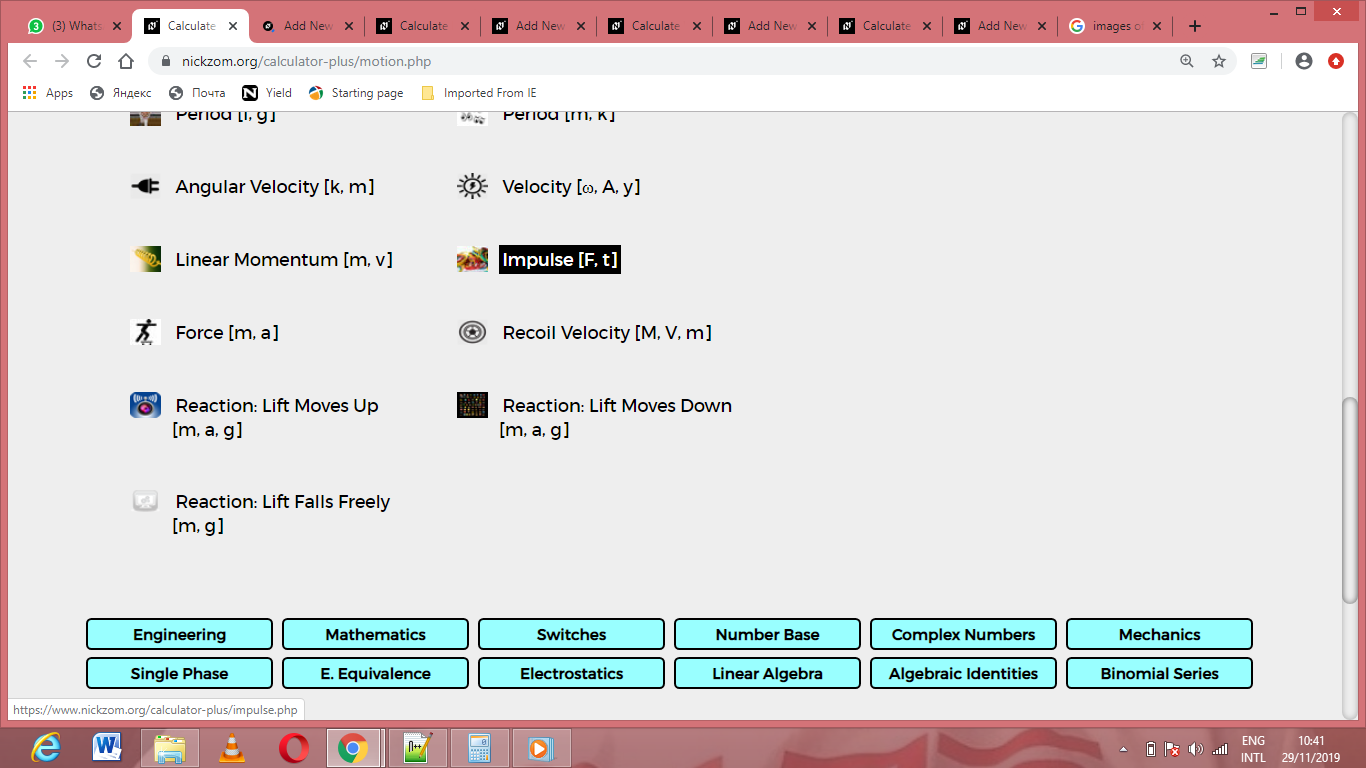The screenshot below displays the page or activity to enter your values, to get the answer for the impulse according to the respective parameters which are the force (f) and time (t).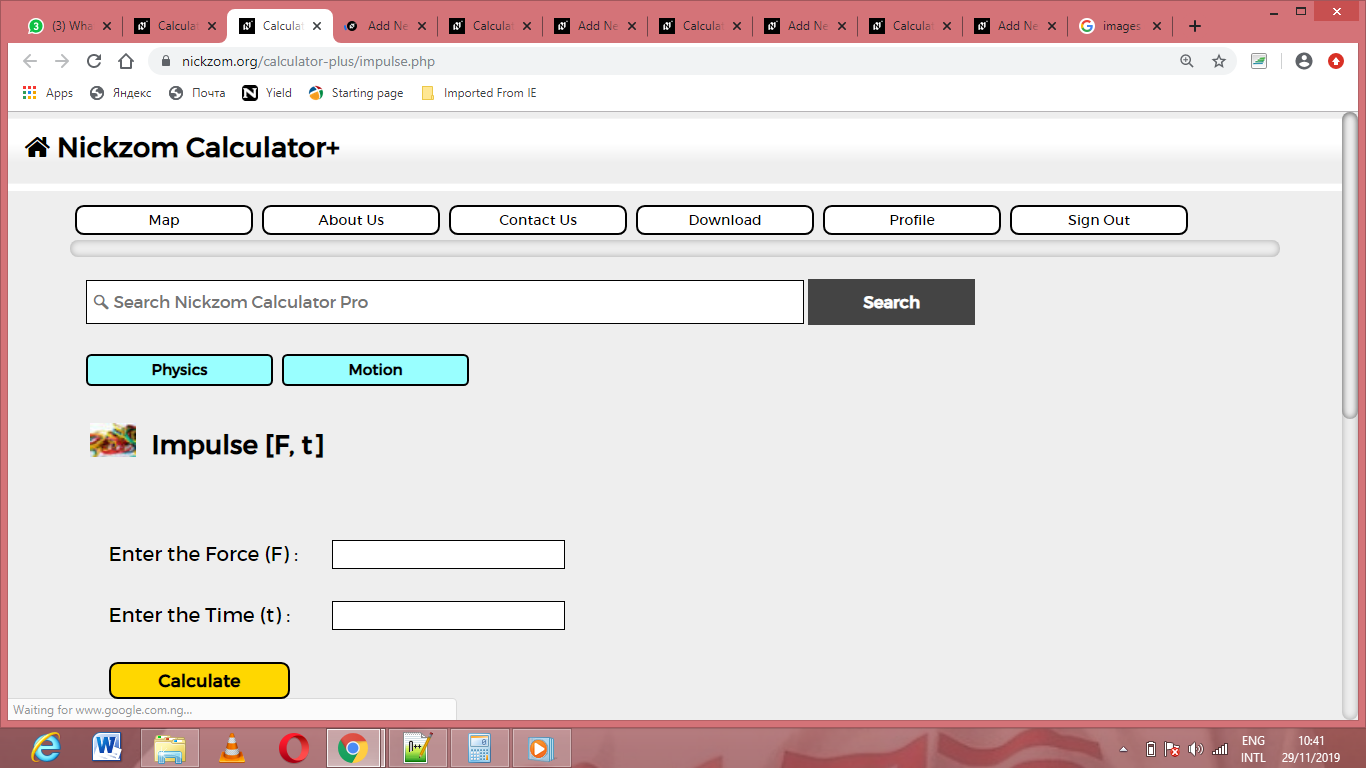Now, enter the values appropriately and accordingly for the parameters as required by the force (f) is 28 and time (t) is 14.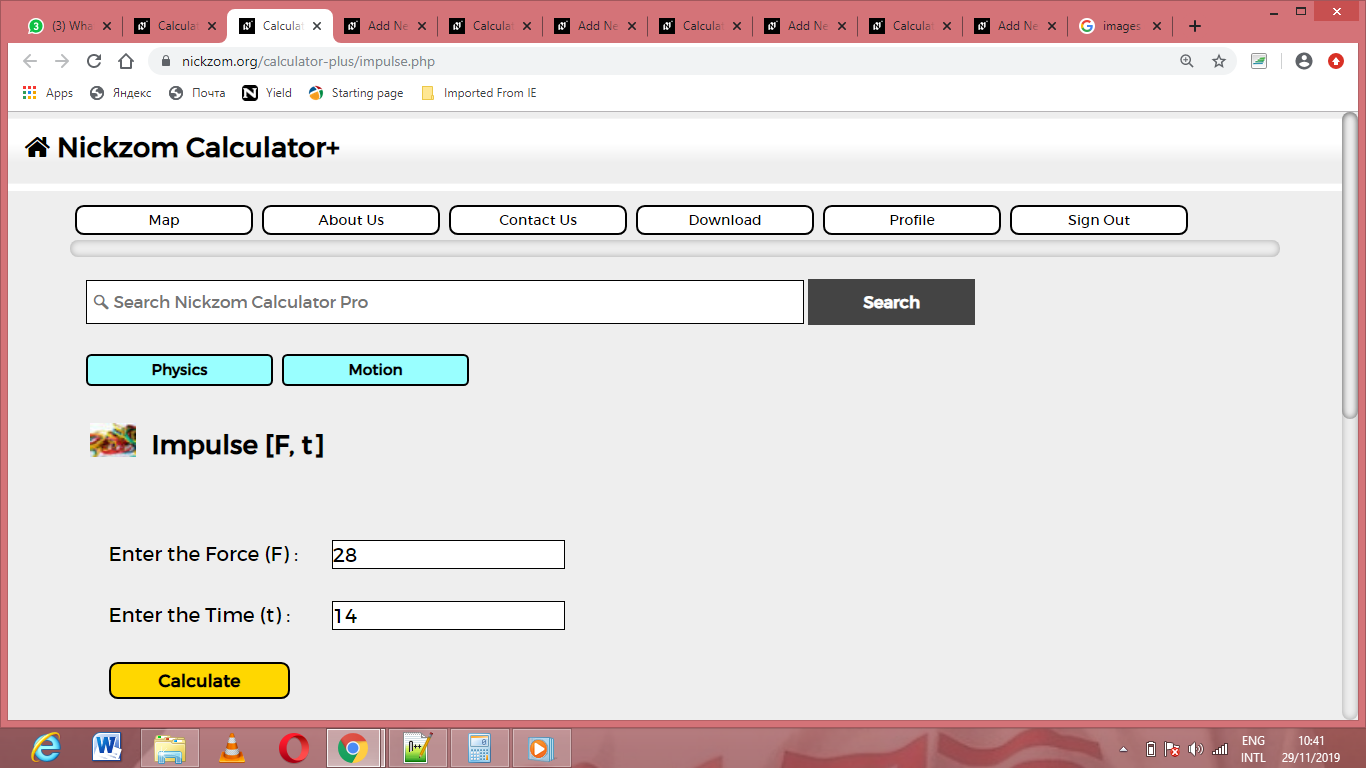Finally, Click on Calculate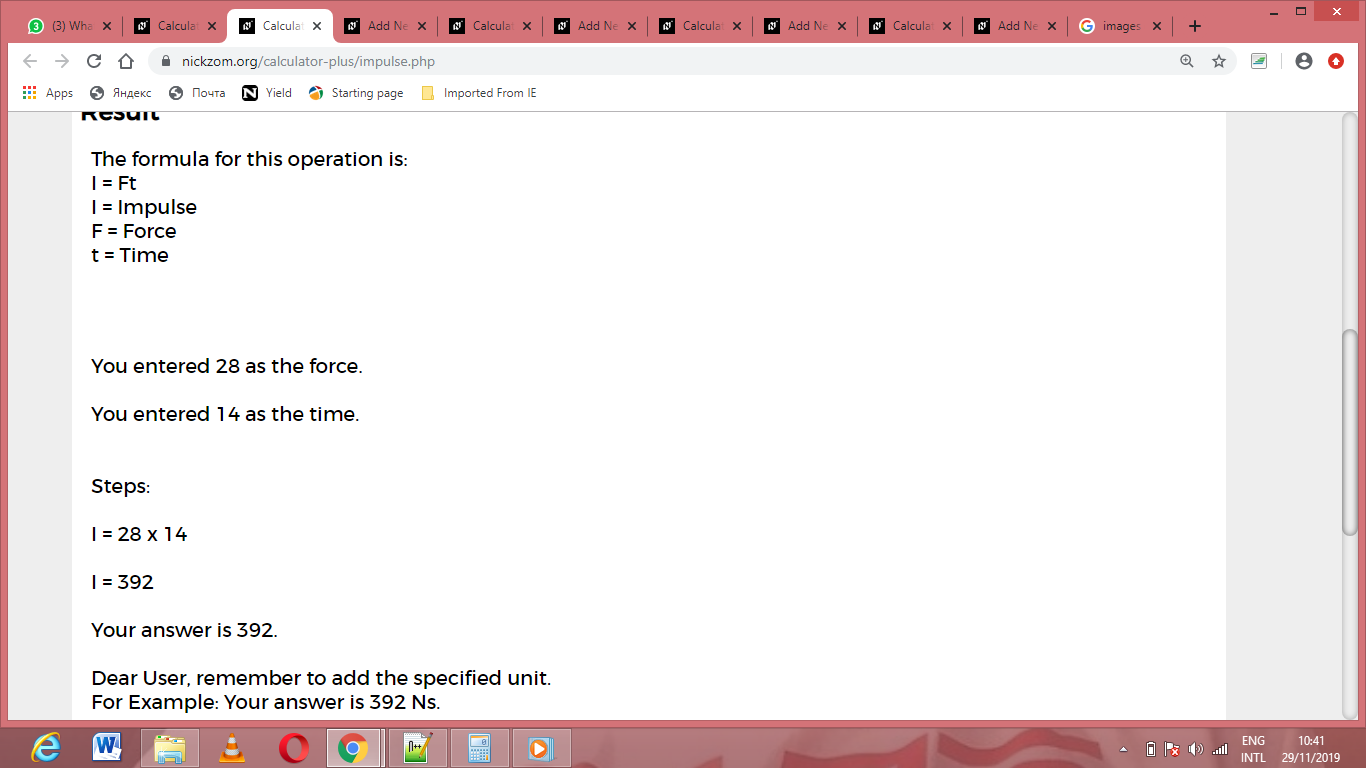As you can see from the screenshot above, Nickzom Calculator– The Calculator Encyclopedia solves for the impulse and presents the formula, workings and steps too.